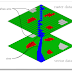GIS stores the information in the form of thematic layers that can be linked together by geography.This presents the complexity off world inn simple form to understand the natural relationships.

GIS DATA TYPES

GIS technology has two basic types of data that it use.These are:

1. SPATIAL DATA

It represents the relative and absolute locations of a geographic feature.

2. ATTRIBUTE DATA

It provides the properties of spatial features. The properties can be qualitative or quantitative. Attribute data is also known as tabular data.
Examples of spatial and attribute data can be seen as: the coordinate location of forestry stand is spatial data while properties of that forestry stand such as dominant species, cover group, crown closure etc are attribute data.Image data may be considered as spatial data e.g. photographs, movies and it may be attribute data e.g. sound, descriptions or narrations etc.

SPATIAL DATA MODELS

Two basic types of spatial data models exist that store geographic data digitally. These are:
• Vector
• Raster
VECTOR DATA FORMATS

All spatial data models are used to store geographic locations of features in database. Geographic features are represented in the form of vectors is represented by vectors (directional lines).
Vector data is represented in the form of Zero Dimensional Objects, One Dimensional Objects and Two Dimensional Objects. Zero dimension objects are Point and Node. Point is defined as a single X,Y coordinate to specify a geometric location. Node is a topological junction that also represents the geometric location. One Dimension Objects are Line Segment, Link, Directed Link, String, Chain, Arc and Ring. Line segment is a direct link between two end points. Link is the connection between two end nodes. Direct link is connection between two nodes with one direction. String is a sequence of line segments. Two dimension objects are Simple Area / Polygon , Complex Area / Polygon , Raster Cell / Pixel.  Simple Area / Polygon is a outer ring that may not have inner ring while complex are/ polygon has outer ring with inner ring as well. Pixel is two dimension object of an element of regular tessellation of a surface.

TYPES OF VECTOR DATA MODELS
•  The Spaghetti  Data Model
•  Topological Data Model
•  Triangular Irregular Data Model (TIN)
•  Shape Files (Arc View, Arc GIS Files)
•  Digital Line Graph (DLG)

Spaghetti  Data Model

In this model point, line and area/polygon concept is given as described earlier. Polygon is a closed loop of coordinates that has its boundary. The common boundary between adjacent polygon is considered two times for each polygon. A file of  spatial data prepared in this way is a collection of strings having coordinates with no inherent structure is known as Spaghetti Data Model. This model is not efficient for spatial analysis.

Topological Data Models

It is mostly used model for encoding spatial relationships in GIS. Topology is defined as a mathematical method to define spatial relationships. It is also called as Arc Node Model.  Arc is a series of points that start and end at a node. While node is a point where two arcs intersects. Dangling Node is a node that is not connected to an other node.

TIN Data Model

Triangular Irregular Network (TIN ) is a Type of vector data model used to describe the terrain data. Triangular sides are used to show terrain. Three vertices X,Y coordinates and Z values are stored against each side. Each triangle has three nodes. Coordinates and topology are stored in different tables. Slope and aspect are measured for every facets. Hardware and software should be more effective to manage large amount of TIN data. It is more accurate than raster models.

RASTER DATA MODELS

Real World in raster data format is represented in the form of square shaped small boxes uniform in size. Locations of geographic features are represented in the form of rows and columns. The area of each cell shows its spatial resolution. A point is represented by a single cell and a line is shown by group of cells combined together. In line each cell has a same attribute value. Each cell has a specific attribute value. Cluster of cells having same value is known as polygon. The small area depicts the higher resolution. Thus larger files are needed to store the data. So, raster cells are larger in size.

GIS DATA TYPES AND DATA MODELSReviewed by Admin on July 05, 2016 Rating: 5